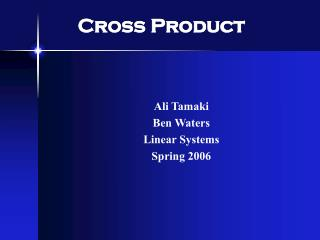DownloadDownload PresentationCross Product

# Cross Product

Download Presentation## Cross Product

- - - - - - - - - - - - - - - - - - - - - - - - - - - E N D - - - - - - - - - - - - - - - - - - - - - - - - - - -
##### Presentation Transcript

1. Cross Product Ali Tamaki Ben Waters Linear Systems Spring 2006

2. Definition The cross product is defined as the vector perpendicular to two given vectors A and B separated by an angle  and is shown by: ||A x B|| = ||A|| ||B|| sin The magnitude of the cross product equals the area of the parallelogram that the initial two vectors span

3. Properties Anti-commutative: a x b = -b x a Distributive over addition: a x (b + c) = a x b + a x c Compatible with scalar multiplication: (ra) x b = a x (rb) = r(a x b) Not associative, but satisfies the Jacobi identity: a x (b x c) + b x (c x a) + c x (a x b) = 0 Cross product is only valid in R3 and R7

4. Solving Methods Given two vectors u = [u1 u2 u3] and v = [v1 v2 v3] u x v = u2v3 – u3v2 u3v1 – u1v3 u1v2 – u2v1

5. Matrix Solving Method The first row is the standard basis vectors and must appear in the order given here. The second row is the components of u [u1 u2 u3] and the third row is the components of v [v1 v2 v3]. Given two vectors u and v, u x v equals the determinant of the matrix.

6. Applications Mathematical Applications Vector Cross Product Geometry Physics Applications Torque Angular Momentum Lorentz Force

7. Vector Cross Product Cross Product Applet ||a x b||= ||a|| ||b||sin() Two non-zero vectors a and b are parallel iff a x b = 0

8. Geometric Applications 3-D Volume of a parallelepiped with sides of length a, b, c equals the magnitude of the scalar triple product: V = |a · (b x c)|

9. Torque & Angular Momentum Torque is the measure of how much a force acting on an object will cause that object to rotate.Ø is T= r x F = r F sin(ø) If a particle with linear momentum p is at a position r with respect to some point, then its angular momentumL is the cross product of r and p L = r xp

10. Torque Example F R • T= R x F = RFsin() • is the angle between the location of the applied force and the point where the radius meets this force. In this case,  = 90, which means that the torque is just the product of the radius and the applied force because sin(90) = 1.

11. Lorentz Force The Lorentz Force F exerted on a charged particle in an electromagnetic field equals the electric charge q of the particle times the electric field E plus the cross product of the velocity v of the particle and the magnetic field B F = q (E + vxB)

12. Lorentz Force RHR Align the thumb of the right hand in the direction of the velocity v. Then, point the index finger of the right hand in the direction of the magnetic field B. Now, the palm of the right hand points in the direction of the Lorentz force F. Lorentz Force Applet

13. Conclusion What do you get when you cross a mountain-climber with a mosquito? Nothing, you can't cross a scalar with a vector.